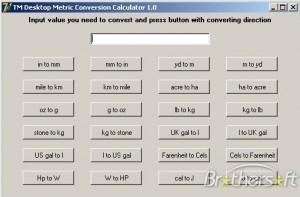# Metric Conversion

There are times when metric conversion will benecessary, such as when you are traveling or conducting research. The following information will explain how to change one measuring scale into the other.

### Length Conversion Guide

When you know inches and want to find millimeters, multiply it by 25.4. If you know feet and want to find out the meter equivalent, multiply the feet by 0.305. Multiply the yards by 0.914 to get meters. To convert miles to kilometers, multiply the miles figure by 1.61.

### Area Conversion Guide

If you know square inches and want to know square millimeters, multiply square inches by 645.2. If you know the square feet and want to find square meters, multiply the square feet by 0.093.

If you know the square yard and want to find the square meters, this metric conversion guide recommends multiplying the sq yd by 0.836. If you know the acre, multiply it by 0.405 and you will find the hectare equivalent. If you know the square miles, multiply it by 2.59 to get square kilometers.

### Volume Conversion Guide

Multiply the fluid ounces by 29.57 to find the milliliter equivalent. To convert from gallons to liters, multiply the gallons by 3.785. Multiply the cubic feet by 0.028 to find the cubic meters. If you know the cubic yards, multiply it by 0.765 to get the cubic meters.

### Mass Conversion Guide

This metric conversion guide suggests multiplying the ounce figure by 28.35 to determine the grams equivalent. To convert pounds to kilograms, multiply pounds by 0.454. If you know the short tons (2000 lb), multiply it by 0.907 to get megagrams (or "metric ton").

Temperature Conversion Guide

The formulas are (F-32) x 5 / 9 and (F-32) / 1.8.

### Illumination Conversion Guide

If you know the foot-candles, multiply it by 10.76 to get the lux equivalent. If you know foot-Lamberts and want the candela/m2 equivalent, multiply the foot-Lamberts figure by 3.426.

### Converting from the Metric System: Length

Multiply millimeters by 0.039 to get inches. Multiply meters by 3.28 to get the feet equivalent. This metric conversion guide suggests multiplying the meter by 1.09 to get yards. To convert from kilometers to miles, multiply kilometers by     0.621.

### Converting from the Metric System: Area

If you know millimeters, multiply it by 0.0016 to get square inches. To convert square meters to square feet, multiply the square meters by 10.764. Multiply square meters by 1.195 to get the square yards equivalent. To convert hectares into acres, multiply hectares by 2.47.

### Converting from the Metric System: Volume and Mass

Multiply milliliters by 0.034 to find the fluid ounces. Multiply liters by 0.264 to get the gallons equivalent. To convert cubic meters to cubic feet, multiply it by 35.314.

You should multiply the cubic meters by 1.307 to get the cubic yard equivalent. To convert grams into ounces, multiply the grams by 0.035. To convert pounds to kilograms, multiply pounds by 0.454.

This metric conversion guide shows only the approximate conversions. Although not exact, these are the figures usually used by people.